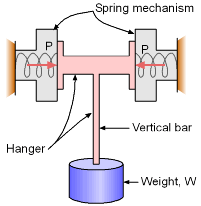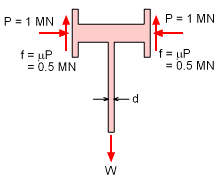Ch 1. Stress and Strain Multimedia Engineering Mechanics NormalStress Shear andBearing Stress NormalStrain Hooke'sLaw ThermalEffects IndeterminateStructures
 Chapter 1. Stress/Strain 2. Torsion 3. Beam Shr/Moment 4. Beam Stresses 5. Beam Deflections 6. Beam-Advanced 7. Stress Analysis 8. Strain Analysis 9. Columns Appendix Basic Math Units Basic Equations Sections Material Properties Structural Shapes Beam Equations Search eBooks Dynamics Fluids Math Mechanics Statics Thermodynamics Author(s): Kurt Gramoll ©Kurt GramollMECHANICS - EXAMPLE ExampleSpring Load Hanging Bracket A "quick release" hanger is designed to carry a weight by using friction. The hanger is suspended by using two spring mechanisms that exert a constant horizontal force P of 1 MN on the two sides of the hanger. The vertical bar that holds the weight is circular and has negligible weight. What is the minimum diameter of this circular bar that can carry the maximum load W? Maximum allowable stress, σ, for the vertical bar material is 100 GPa and coefficient of friction, μ, between sides of hanger and rough surface of spring mechanism is 0.5. SolutionFree-Body Diagram The spring mechanisms exert a horizontal force P of 1 MN. This force generates friction, f at both sides of the hanger.      f = μP        = (0.5)(1 MN)        = 0.5 MN Since, the whole hanger system is suspended only by this frictional force, it can carry a maximum weight, W of      W = 2f = 2(0.5) = 1 MN Ignoring the weight of the hanger, the minimum cross sectional area, A, of the vertical part, is      σ = F/A      100,000 MPa = 1.0 MN / A      A = 1.0×10-5 m2         = πr2 = πd2/4 Corresponding diameter of the rod,      d = 3.568 ×10-3 m         = 3.568 mm

Practice Homework and Test problems now available in the 'Eng Mechanics' mobile app
Includes over 400 problems with complete detailed solutions.
Available now at the Google Play Store and Apple App Store.